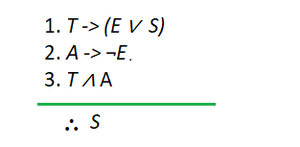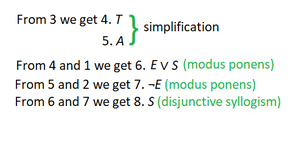GeeksforGeeks App
Open AppBrowser
Continue

# Discrete Mathematics – Applications of Propositional Logic

A proposition is an assertion, statement, or declarative sentence that can either be true or false but not both. For example, the sentence “Ram went to school.” can either be true or false, but the case of both happening is not possible. So we can say, the sentence “Ram went to school.” is a proposition. But, the sentence “N is greater than 100” is not a proposition as we cannot state that whether it is true or false unless the value of N is given. A few more examples of propositions are “12 – 10 = 3”, “The library is open.”, etc.

The area of mathematical logic that deals with propositions are called Propositional Logic or Propositional Calculus. It is also known as sentential logic or sentential calculus. It studies the logical values of propositions when taken as a whole and logical relationships when connected using logical connectives(such as logical AND, logical OR, etc.).

### Importance of Propositional Logic

Propositional Logic plays an important role in computer science as well as in a person’s daily life. The main benefits of studying and using propositional logic are that it prevents us from making inconsistent inferences and incautious decisions. It incorporates reasoning and thinking abilities in one’s daily life.

### Applications of Propositional Logic

In the computer science field, propositional logic has a wide variety of applications and hence is very important. It is used in system specifications, circuit designing, logical puzzles, etc. Apart from this, it can also be used in translating English sentences to mathematical statements and vice-versa. Let us look at this vast variety of applications in detail.

#### 1) Translating English Sentences into logical statements

Like any other human language, English sentences can be ambiguous. This ambiguity might lead to uninformed decision-making and other fatal errors. To remove this ambiguity, we can translate these English sentences into logical expressions with the help of Propositional Logic. Note that sometimes this may include making a few assumptions based on the sentence’s intended meaning.

Example: Given a sentence “You can purchase this book if you have \$20 or \$10 and a discount coupon.” Now, this is a bit complex to be understood at once. So we translate this into a logical expression that will make it simple to understand. Let a, b, c, and d represent the sentences “You can purchase this book.“, “You have \$20.“, “You have \$10.“, and “You have a discount coupon.” respectively. Then the given sentence can be translated to  (b ∨ (c ∧ d) -> a, which simply means that “if you either have \$20 or \$10, along with a discount coupon, then you can purchase the book.”

#### 2) System Specifications

When developing/manufacturing a system(Software or Hardware), the developers/manufacturers have to meet certain needs and specifications, which are usually stated in English. But as English sentences can be ambiguous, developers/engineers translate these system specifications into logical expressions to state specifications rigorously and unambiguously.

Example: Let a, b, c, and d represent the sentences “The computer is within the local network.“, “The computer has a valid login id.“, “The computer is under the use of administrator.“, and “Internet is accessible to the computer.” So the complex sentence, “If the computer is within the local network or it is not within the local network but has a valid login id or it is under the use of administrator, then the Internet is accessible to the computer.” can be expressed as (a ∨ (¬a ∧ b) ∨ c) -> d.

#### 3) Logical Puzzles

Puzzles that are solved using reasoning and logic are called logical puzzles. They can be used for brain exercises, recreational purposes, and for testing a person’s reasoning capabilities. Solving such puzzles is generally tricky, but it can be done easily using propositional logic. Some of the famous logic puzzles are the muddy children puzzle, Smullyan’s puzzles about knights and knaves, etc.

Example:

Problem Statement: There is an island that has two kinds of inhabitants, knights, who always tell the truth, and their opposites, knaves, who always lie. You encounter two people A and B. Determine what are A and B if A says “B is a knave” and B says “Both of us are of same types”?

Solution: Let p and q be the statements that A is a knight and B is a knight, respectively, so ¬p and ¬q are the statements that A is a knave and B is a knave, respectively. Let’s assume A is a knight, i.e., p is true. So A is telling the truth, which means ¬q is true. Now as B is a knave whatever it is saying is a lie, i.e., (p ∧ q) ∨ (¬p ∧ ¬q) is false, which simply means if either of them is a knave then the other one is a knight or vice-versa. Now, as per the assumption, this statement is true. So we can conclude that A is a knight and B is a knave.

#### 4) Boolean Searches

Another important application of propositional logic is Boolean searches. These searches use techniques from propositional logic. Logical connectives are used extensively in searches of large collections of information, such as indexes of Web Pages. In Boolean searches, the connective AND is used to find records that contain both of the two terms, the connective OR is used to find records that have either one or both of the terms, and the connective NOT, also written as AND NOT, is used when we need to exclude a particular search term.

Example: Some web search engines support web page searching that uses Boolean techniques. For instance, if we want to look for web pages about hiking in West Alps, then we can look for pages matching WEST AND ALPS AND HIKING. And if we want web pages about hiking in the Alps, but not in West Alps, then we can search for pages matching the record HIKING AND ALPS AND NOT(WEST AND ALPS).

#### 5) Logic/Computer Circuits

Logic gates or circuits are electronic devices that implement Boolean functions, i.e. it does a logic operation on one or more bits of input and gives a bit as an output. They are the basic building blocks of any digital system. The relationship between the input and output is based on a certain propositional logic.

Example: Logic gates are named as AND gate, OR gate, NOT gate, etc. based on the names of logical connectives AND, OR, NOT, etc. The output truth values for the given input bits for these gates are the same as that those returned by the logical connectives.

#### 6) Inference and Decision Making

Propositional Logic is widely used in the making rules of inference and decision making. These rules of inferences can then be used to build arguments. When several premises are given, it is hard to tell if a given argument is valid. Thus, we use these rules of inference to validate an argument and make a decision.

Example: We can prove that the following premises build up a valid argument using the rule of inference.

If today is Tuesday, I have a test in English or Science. If my English Professor is absent, then I will not have a test in English. Today is Tuesday and my English Professor is absent. Therefore I have a test in Science.

T: Today is Tuesday

E: I have a test in English

S: I have a test in Science

A: My English Professor is absentPremises as logical notationsRules of Inference

Hence, the argument stated is valid.

#### 7) Artificial Intelligence – Fuzzy Logic

AI algorithms make use of fuzzy logic. In fuzzy logic,  there is no logic for the absolute truth and absolute false value. But in fuzzy logic, there is an intermediate value too present which is partially true and partially false.

For Example: The truth value 0.6 can be assigned to the statement “Fred is happy”, because Fred is happy slightly more than most of the time, and the truth value 0.5 can be assigned to the statement “Percy is happy” because Percy is happy half of the time.

My Personal Notes arrow_drop_up